# Difference between revisions of "Uniqueness theorem for limits"

## Statement

### Two-sided limit

Suppose$f$ is a function and$c$ is a point such that$f$ is defined on both the immediate left and the immediate right of$c$. The uniqueness theorem for limits states that if the limit of$f$ exists at$c$ (in the sense of existence as a finite real number) then it is unique. In other words:

If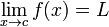$\lim_{x \to c} f(x) = L$ and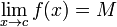$\lim_{x \to c} f(x) = M$, then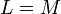$L = M$.

### Left hand limit

Suppose$f$ is a function and$c$ is a point such that$f$ is defined on the immediate left of$c$. The uniqueness theorem for left hand limits states that if the left hand limit of$f$ exists at$c$ (in the sense of existence as a finite real number) then it is unique. In other words:

If$\lim_{x \to c^-} f(x) = L$ and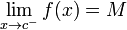$\lim_{x \to c^-} f(x) = M$, then$L = M$.

### Right hand limit

Suppose$f$ is a function and$c$ is a point such that$f$ is defined on the immediate right of$c$. The uniqueness theorem for right hand limits states that if the right hand limit of$f$ exists at$c$ (in the sense of existence as a finite real number) then it is unique. In other words:

If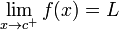$\lim_{x \to c^+} f(x) = L$ and$\lim_{x \to c^+} f(x) = M$, then$L = M$.